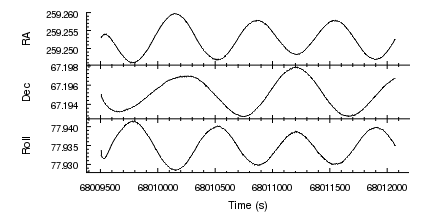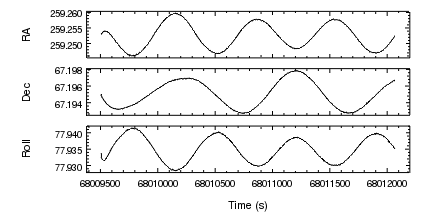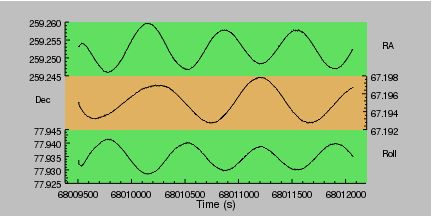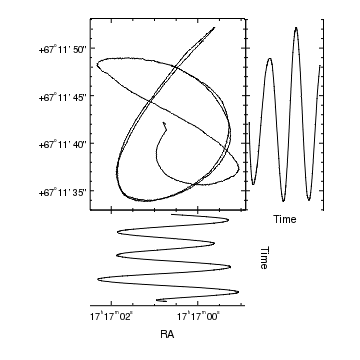Gallery: Sharing an axis between plots

1) Three datasets in separate plots with a common axis

There are a several ways to create a grid of plots; in this example we use the strip_chart command to create three vertically-aligned plots which have a "shared" or "common" X axis (so that changes to the scaling or limits of one X axis is automatically reflected in all three plots). It also highlights some of the different ways to control the appearance of axes; in particular how to select the arrangement of major and minor tick marks.

The data to be plotted is from the aspect solution of a Chandra observation; we wish to show how the RA, Dec, and Roll of the telescope varied with time.Version: Postscript; PDF
```pref = ChipsPreferences()
pref.curve.symbol.style = "none"
pref.plot.style = "open"
pref.axis.offset.perpendicular = 70
pref.axis.ticklabel.offset = 10
pref.plot.leftmargin = 0.2
pref.plot.bottommargin = 0.2
pref.plot.rightmargin = 0.05
pref.plot.topmargin = 0.05
set_preferences(pref)

strip_chart(3)

ax = ChipsAxis()
ax.majortick.style = "centered"
ax.minortick.style = "centered"
ax.majortick.length = 8
ax.minortick.length = 4
set_xaxis(ax)
set_yaxis(["majortick.interval",5e-3,"tickformat","%.3f"])
set_plot_ylabel("RA")

current_plot("plot2")

# We reuse the settings from the first plot for the X axis
set_xaxis(ax)
set_yaxis(["majortick.interval",2e-3,"tickformat","%.3f"])
set_plot_ylabel("Dec")

current_plot("plot3")

set_plot_xlabel("Time (s)")
set_plot_ylabel("Roll")
set_xaxis(["tickformat","%.0f","offset.perpendicular",45])
set_yaxis(["majortick.interval",5e-3,"tickformat","%.3f"])
```

Before we create the plot we change several ChIPS preferences: curves are only displayed as lines, plots are drawn using the "open" style - which means that only the bottom and left edges of the plot contain an axis, the plot margins are changd to better make use of the space in the frame given the data being plotted, and the offsets for the axis labels are increased. The set_preferences call applies these settings.

Since the default window size is square, the add_window routine is used to create a rectangular window. We then fill this window with three vertically-arranged plots by calling strip_chart. At this point the output of info and info_current looks like:

```chips> info()

Window [win1]
Frame [frm1]
Plot [plot1]   (0.20,0.70)  .. (0.95,0.95)
Border bottom [bx1]  top [bx2]  left [by1]  right [by2]
X Axis [ax1]
Y Axis [ay1]
Plot [plot2]   (0.20,0.45)  .. (0.95,0.70)
Border bottom [bx1]  top [bx2]  left [by1]  right [by2]
X Axis [ax1]
Y Axis [ay1]
Plot [plot3]   (0.20,0.20)  .. (0.95,0.45)
Border bottom [bx1]  top [bx2]  left [by1]  right [by2]
X Axis [ax1]
Y Axis [ay1]

chips> info_current()

Window [win1]
Frame [frm1]
Plot [plot1]
X Axis [ax1]
Y Axis [ay1]
Coord Sys [Data]
Coord Sys ID [ds0.0.0.6]

```

The top plot, which is current, is used to display the RA versus time data. The tick marks for both axes in this plot are adjusted using the set_xaxis and set_yaxis calls; of note is the change in the majortick.interval setting which determines the spacing of the major tick marks.

The second plot is used to display the variation of the telescope Declination with time and the third plot the roll variation. In all plots similar adjustments are made to tweak the axes.

2) Three datasets in separated plots

Here we plot the same data as the initial example, this time with a small vertical gap between the plot created by setting the optional third argument of strip_chart to 0.05 (the default is 0).Version: Postscript; PDF
```set_preferences(["curve.symbol.style","none","axis.offset.perpendicular",70])
set_preferences(["plot.leftmargin",0.2,"plot.bottommargin",0.2])
set_preferences(["plot.rightmargin",0.05,"plot.topmargin",0.05])

strip_chart(3,0,0.05)

set_yaxis(["majortick.interval",5e-3,"tickformat","%.3f"])
set_plot_ylabel("RA")

current_plot("plot2")

set_yaxis(["majortick.interval",2e-3,"tickformat","%.3f"])
set_plot_ylabel("Dec")

current_plot("plot3")

set_plot_xlabel("Time (s)")
set_plot_ylabel("Roll")
set_xaxis(["offset.perpendicular",45,"tickformat","%.0f"])
set_yaxis(["majortick.interval",5e-3,"tickformat","%.3f"])
```

In both this visualization and the previous example, the preferences for the plot margins were changed before strip_chart was called. This is because the margin preferences are used to layout the plots, including calls used to adjust the spacing between the plots or their relative sizes, not the values used by the current plot.

3) Three datasets in separate plots with a hidden X axis

Here we take the arrangement from the first example and hide the X axes of the top two plots to make it appear as if there is a single plot with three different Y axes.Version: Postscript; PDF
```pref = ChipsPreferences()
pref.curve.symbol.style = "none"
pref.plot.style = "open"
pref.axis.offset.perpendicular = 30
pref.plot.rightmargin = 0.15
set_preferences(pref)

strip_chart(3)

# Set up the axis and labels
hide_axis("ax1")
set_yaxis(["majortick.count",4,"tickformat","%.3f"])

current_plot("plot2")

# Hide the default X (bottom) and move the Y axis to display on the right
hide_axis("ax1")
move_axis("ay1",1,0)
set_yaxis(["majortick.count",4,"tickformat","%.3f"])

# Add a label where the Y axis used to be.

current_plot("plot3")

set_xaxis(["tickformat","%.0f"])
set_yaxis(["majortick.count",5,"tickformat","%.3f"])

set_plot_xlabel("Time (s)")

# Add a region, in plot-normalized coordinates, behind each plot,
# to act as a background.
xr = [0, 1, 1, 0]
yr = [0, 0, 1, 1]
current_plot("all")
current_plot("plot2")
set_region(["fill.color","orange"])

# Now set the frame background
set_frame(["bgcolor","gray"])
```

In the middle plot we want to hide both the X and Y axes and display a Y axis on the right-hand side. This can be done by moving the Y axis (called "ay1") to the right-side of the plot using move_axis. As the axis was created before any data was added to the plot, it was placed using the plot-normalized coordinate system, so the coordinates used to move the axis should also be in this system: here we move the axis to x=1 which corresponds to the right-side of the plot (x=0 is the left side of the plot area).

The set_yaxis call recognizes that setting majortick.count means that the majortick.mode setting should also be changed to count.

The approach used to highlight each plot by giving it a different background color is explained in the adding a background to a plot example. Since the regions are defined in the plot-normalised coordinate system they will always fill the plot, even if their sizes are changed by calls like

```adjust_grid_ygap(0.05)
```

4) A grid where the plots share axes and the plot sizes are different

The split command is useful when you want to create a grid of plots (i.e. with multiple rows and columns). In this example we use it to create a 2 by 2 arrangement of plots; this is then modified by deleting the last plot and adjusting the relative widths and heights so that the first plot is twice as big as the other two plots.

The main plot is used to show the Right Ascension and Declination of the telescope varied together (the data used is a small subset of the Chandra observation and so does not show the full Lissajous curve you would expect to see). The secondary plots are used to show the temporal variation of the two axes; even though the time axes in these plots have been hidden for display purposes, you can still display data using these axes.Version: Postscript; PDF
```pref = ChipsPreferences()
pref.curve.symbol.style = "none"
pref.plot.leftmargin = 0.25
pref.plot.rightmargin = 0.1
pref.plot.topmargin = 0.05
set_preferences(pref)

split(2,2)

# Hide the X axis
set_xaxis(["ticklabel.visible",False])

ay = ChipsAxis()
ay.majortick.interval = 2e-3
ay.minortick.count = 3
ay.tickformat = "dec"
set_yaxis(ay)

# Move to the top-right plot and show the
# time variation of the Declination
current_plot("plot2")

# Make the Y axis the same as the first plot
bind_axes("plot1","ay1","plot2","ay1")

# Hide the numeric labels on both axes
set_axis(["ticklabel.visible",False])

# Label the right-hand Y axis
set_axis("by2",["y.label","Dec","offset.perpendicular",10])

# Hide both the lower and upper X axes

# Add a label below the plot along the X axis
# (we can not add it to the X axis since it is hidden,
# so we position it using the plot-normalized coordinate
# system to ensure it is centered horizontally and
# placed below the plot)

# Remove the fourth plot created by split
delete_plot("plot4")

# Move to the bottom-left plot and show the
# time variation of RA, but with time as the
# ordinate.
current_plot("plot3")

# Make the X axis the same as the first plot
bind_axes("plot1","ax1","plot3","ax1")

set_plot_xlabel("RA")
set_xaxis(["tickformat","ra"])
set_xaxis(["majortick.interval",1e-2,"minortick.count",3])

# Since the X axes of plot1 and plot3 are bound together,
# this changes the display of both plots.
reverse_axes(X_AXIS)

# Hide both the left and right Y axes

# Add a label to the right of the plot along the Y axis
```

For full control over the grid - including support for varying number of rows or columns, use one of the the grid_objects, col_grid_objects, or row_grid_objects routines.

The info output for this visualization is:

```Window [win1]
Frame [frm1]
Plot [plot1]   (0.25,0.37)  .. (0.68,0.90)
Border bottom [bx1]  top [bx2]  left [by1]  right [by2]
Curve [crv1]
X Axis [ax1]
Y Axis [ay1]
Plot [plot2]   (0.68,0.37)  .. (0.90,0.90)
Border bottom [bx1]  top [bx2]  left [by1]  right [by2]
Curve [crv1]
X Axis [ax1]
Y Axis [ay1]
Label [lbl1]
Plot [plot3]   (0.25,0.10)  .. (0.68,0.37)
Border bottom [bx1]  top [bx2]  left [by1]  right [by2]
Curve [crv1]
X Axis [ax1]
Y Axis [ay1]
Label [lbl1]
```